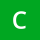Home
IT Knowledge
Inspiration
Languages
EN

# Python - replace part of string from given index

0 points
Created by:Chronal
307

In this article, we would like to show you how to replace part of string from given index in Python.

Quick solution:

``````text = "ABCD"
replacement = "xy"

position = 1
number_of_characters = 2

result = text[:position] + replacement + text[position + number_of_characters:]

print(result)  # AxyD``````

## 1. Practical example using string slicing with string concatenation

In this example, we use string slicing with string concatenation to replace `CD` letters (index 1-3) from the `text` with the `replacement`.

``````text = "ABCD"
replacement = "xy"

position = 1
number_of_characters = 2

result = text[:position] + replacement + text[position + number_of_characters:]

print(result)  # AxyD``````

Output:

``AxyD``

Explanation:

piece of codeequivalent toletter
`text[:position]``text[0:1]`A
`text[position + number_of_characters:]``text[3:text.length]` or `text[3:4]`D

## 2. Practical example using string slicing with `join()` method

In this example, we use string slicing with `join()` method to replace `CD` letters (index 1-3) from the `text` with the `replacement`.

``````text = "ABCD"
replacement = "xy"

position = 1
number_of_characters = 2

result = "".join((text[:position], replacement, text[position + number_of_characters:]))

print(result)  # AxyD``````

Output:

``AxyD``